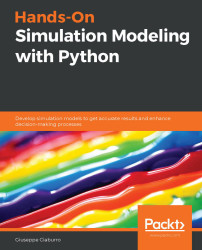•#### Hands-On Simulation Modeling with Python#### Overview of this book

Simulation modeling helps you to create digital prototypes of physical models to analyze how they work and predict their performance in the real world. With this comprehensive guide, you'll understand various computational statistical simulations using Python. Starting with the fundamentals of simulation modeling, you'll understand concepts such as randomness and explore data generating processes, resampling methods, and bootstrapping techniques. You'll then cover key algorithms such as Monte Carlo simulations and Markov decision processes, which are used to develop numerical simulation models, and discover how they can be used to solve real-world problems. As you advance, you'll develop simulation models to help you get accurate results and enhance decision-making processes. Using optimization techniques, you'll learn to modify the performance of a model to improve results and make optimal use of resources. The book will guide you in creating a digital prototype using practical use cases for financial engineering, prototyping project management to improve planning, and simulating physical phenomena using neural networks. By the end of this book, you'll have learned how to construct and deploy simulation models of your own to overcome real-world challenges.
PrefaceSection 1: Getting Started with Numerical SimulationFree Chapter
Chapter 1: Introducing Simulation ModelsChapter 2: Understanding Randomness and Random NumbersChapter 3: Probability and Data Generation ProcessesSection 2: Simulation Modeling Algorithms and TechniquesChapter 4: Exploring Monte Carlo SimulationsChapter 5: Simulation-Based Markov Decision ProcessesChapter 6: Resampling MethodsChapter 7: Using Simulation to Improve and Optimize SystemsSection 3: Real-World ApplicationsChapter 8: Using Simulation Models for Financial EngineeringChapter 9: Simulating Physical Phenomena Using Neural NetworksChapter 10: Modeling and Simulation for Project ManagementChapter 11: What's Next?Other Books You May Enjoy# Classifying simulation models

Simulation models can be classified according to different criteria. The first distinction is between static and dynamic systems. So, let's see what differentiates them.

## Comparing static and dynamic models

Static models are the representation of a system in an instant of time, or representative models of a system in which the time variable plays no role. An example of a static simulation is a Monte Carlo model.

Dynamic models, on the other hand, describe the evolution of the system over time. In the simplest case, the state of the system at time t is described by a function x (t). For example, in population dynamics, x (t) represents the population present at time t. The equation that regulates the system is dynamic: it describes the instantaneous variation of the population or the variation in fixed time intervals.

## Comparing deterministic and stochastic models

A model is deterministic when its evolution, over time, is uniquely determined by its initial conditions and characteristics. These models do not consider random elements and lend themselves to be solved with exact methods that are derived from mathematical analysis. In deterministic models, the output is well determined once the input data and the relationships that make up the model have been specified, despite the time required for data processing being particularly long. For these systems, the transformation rules univocally determine the change of state of the system. Examples of deterministic systems can be observed in some production and automation systems.

Stochastic models, on the other hand, can be evolved by inserting random elements into the evolution. These are obtained by extracting them from statistical distributions. Among the operational characteristics of these models, there is not just one relationship that fits all. There's also probability density functions, which means there is no one-to-one correspondence between the data and system history.

A final distinction is based on how the system evolves over time: this is why we distinguish between continuous and discrete simulation models.

## Comparing continuous and discrete models

Continuous models represent systems in which the state of the variables changes continuously as a function of time. For example, a car moving on a road represents a continuous system since the variables that identify it, such as position and speed, can change continuously with respect to time.

In discrete models, the system is described by an overlapping sequence of physical operations, interspersed with inactivity pauses. These operations begin and end in well-defined instances (events). The system undergoes a change of state when each event occurs, remaining in the same state throughout the interval between the two subsequent events. This type of operation is easy to treat with the simulation approach.

Important Note

The stochastic or deterministic, or continuous or discrete, nature of a model is not its absolute property and depends on the observer's vision of the system itself. This is determined by the objectives and the method of study, as well as by the experience of the observer.

Now that we've analyzed the different types of models in detail, we will learn how to develop a numerical simulation model.